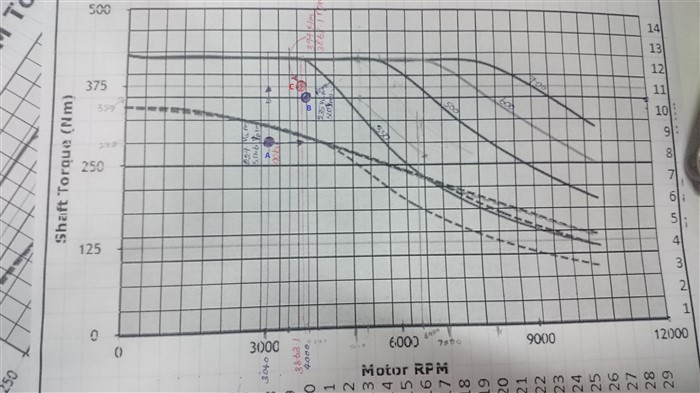If you have a related question, please click the "Ask a related question" button in the top right corner. The newly created question will be automatically linked to this question.

• Resolved

# Motor performance mismatch with the datasheet

Hi yanming and all,

We are using Lab5a with the resolver feedback. Electrical angle calculation is based on TI resolver to digital converter kit hardware and firmware.

We run the motor at 227Vrms(line to line) and 531Arms(phase). At that time motor torque was 289Nm(shaft output) and 3040rpm. But according to the datasheet of the motor, there should be 377Nm, 3863rpm at that time by considering torque constant and the backemf constant. We have a loss of nearly 50kW.

But we cannot increase rpm or line voltage further (Seems we have hit the base rpm according to battery voltage 305Vdc at that time).
We have tested data from the motor manufacture. They have run the motor with 355Nm,4000rms output at 235Vrms(line to line) and 500Arms(phase).

Point A(blue) - Current operating Point (227Vrm,531Arm)

Point C (red) -  Operating point of point A, 227Vrm,531Arm according to motor datasheet. ( calculated using motor constants ke and kt)

Point B (blue) - tested data from motor manufacture for 235Vrms, 500Arms

What will be the reason for this mismatch?Thank you

• Hi Lakshan,

It seems like the MCU is feeding the motor with the necessary voltage/current so i assume there is either a mismatch in the angle information from the resolver or the control loop is not configured correctly.

Best regards,

Simon Jagla

• In reply to Simon Jagla:

Hi Simon,

Thank you for the answer. We changed the angle offset and got the 330Nm for 500Arms. It's a good result with comparatively previous one. But still we are in behind around 20Nm to reach datasheet performance. That was the maximum torque output we could get for 500Arms and that was the best angle offset we could find. We did some Q-axis alignment practicals to find that (forced electrical angle to zero and put d-axis current). Still I have few questions.
What is the best method to find electrical angle offset accurately?
Are there any method to do angle delay compensation of resolver angle when the motor is running at higher rpms?

We are using a PMSM motor with 600Arms/ 700V maximum rating. It is a 10 pole motor.
Thank you

Hi Simon,

We used resolver offset angle 0.96 to get the maximum torque output for the forward direction. But when we tried the same in reverse direction, the torque output is around 10% (very small amount).

What may be the course for this?

How do you define this offset? do you have to consider the direction when defining the offset? Did you check what the correct offset value is for the negative direction?

For me its hard to say at this point but i still assume that the alignment is the issue.

Best regards,

Simon Jagla

• In reply to Simon Jagla:

We are using resolver to digital converter kit based HW and SW to get the angle from resolver. As you know the output of the rslvrOut.angleRaw20 is -1 to +1 in pu scale. As far as I know that angle represent -180 to +180 (correct me if it is wrong).

Our resolver is 5X one and the motor is also having 5 pole pairs. Then it directly produce electrical angle. Other steps are  as below,

// Set pu angle data to 0 - 360 range from -180 to 180

if(rslvrOut.angleRaw20 >= _IQ20(0.0)){

resolver_electrical_angle = rslvrOut.angleRaw20;

}else{

resolver_electrical_angle = rslvrOut.angleRaw20 + _IQ20(2.0);

}

if(resolver_electrical_angle < _IQ20(0.0)){resolver_electrical_angle = _IQ20(0.0);}

else if(resolver_electrical_angle > _IQ20(2.0)){resolver_electrical_angle = _IQ20(2.0);}

resolver_electrical_angle_scalled = resolver_electrical_angle/2;

// Set initial angle offset

motor_electrical_angle = resolver_electrical_angle_scalled - initial_angle_offset;

if(motor_electrical_angle < _IQ20(0.0)){motor_electrical_angle = motor_electrical_angle + _IQ20(1.0) ;}

else {motor_electrical_angle = motor_electrical_angle;}

Is there any initial angle difference for forward and reverse direction?